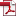## The Postulates of Special Relativity (1)

The two postulates in Special Relativity are stated like this in "On the Electrodynamics of Moving Bodies" :

1. The laws by which the states of physical systems undergo change are not affected, whether these changes of state be referred to the one or the other of two systems of co-ordinates in uniform translatory motion.
2. Any ray of light moves in the "stationary" system of co-ordinates with the determined velocity c, whether the ray be emitted by a stationary or by a moving body.

In "Relativity: The Special and General Theory", Albert Einstein explained these two postulates in detail. Let's first try by ourselves to see if we can understand them, and then in a later section, we'll check the explanations given by Einstein.

The first postulate is the principle of relativity. It says that any physical law is not affected by the selection of reference frames, as far as these reference frames are all in uniform motion.

In the field of mechanical movement, the principle of relativity is shown like this: Newton’s second law of motion, F=ma, works exactly the same in all reference frames. A short mathematical proof is given below.